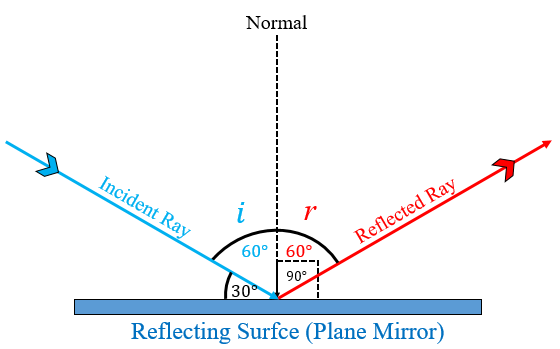# The angle between an incident ray and the plane mirror is 30°. The total angle between the incident ray and reflected ray will be:(a) 30° (b) 60° (c) 90° (d) 120°

(d) 120°

Explanation

Given,

The angle between an incident ray and the plane mirror = 30°

Then, the angle of incidence (angle between an incident ray and the normal) = 90° $-$ angle between the incident ray and plane mirror = 9 $-$ 30° = 60°

Now, according to the first law of reflection, Angle of incidence (∠i) = Angle of reflection (∠r) = 60°

Thus, the total angle between the incident ray and reflected ray = 60° $+$ 60° = 120°.Updated on: 10-Oct-2022

1K+ Views